## Collocation BEM for 3D Poisson Equation

Download CBEM_POI, a package for solving the 3D Poisson equation based on a piecewise constant Collocation Boundary Element Method, and employing a boundary-only discretization technique.

# Piecewise constant collocation method

The functions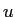and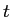are assumed to be constant over each boundary element. It can be shown (see cbem_poiGuide.pdf provided in the package CBEM_POI) that the potentials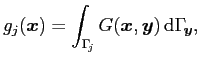and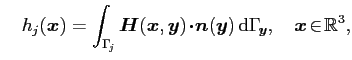due to a uniform source distribution over a flat triangle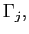can be employed to successfully computeandon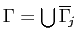. In addition, these potentials can be utilized to effectively calculateat interior points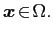The analytic expressions for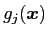and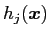over a flat triangle are given in . Moreover, due to its boundary representation, the Newton potential can also be effectively evaluated without the need of a volume-fitted mesh . A brief description of the boundary-only discretization of the Newton potential can be found in the user guide cbem_poiGuide.pdf provided in the package CBEM_POI.

# References

 S. Nintcheu Fata.
Explicit expressions for 3D boundary integrals in potential theory.
Int. J. Num. Meth. Eng., 78(1):32-47, 2009.
 S. Nintcheu Fata.
Treatment of domain integrals in boundary element methods.
Appl. Num. Math., 62(6):720-735, 2012.